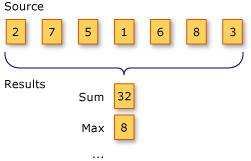#2018 iT 邦幫忙鐵人賽DAY 28
1
Software Development

## 功能說明• `Source`是一個數字陣列
• `Results`有兩種: `Sum``Max`
• `Sum`: 加總`Source`的元素數值
• `Max`: `Source`元素數值的最大值

## 方法定義

`Aggregate`三個公開方法，我們由單純到複雜來說明:

### 第一個方法

``````public static TSource Aggregate<TSource>(
this IEnumerable<TSource> source,
Func<TSource, TSource, TSource> func);
``````
• `func`: 彙整資料所做的處理函式
• `func`第一個傳入參數: 之前元素的彙整結果
• `func`第二個傳入參數: 目前元素的資料
• `func`的回傳值: 之前元素跟目前元素的彙整結果

### 第二個方法

``````public static TAccumulate Aggregate<TSource, TAccumulate>(
this IEnumerable<TSource> source,
TAccumulate seed,
Func<TAccumulate, TSource, TAccumulate> func);
``````
• `seed`: 初始的累加值
• `func`: 彙整資料所做的處理函式

### 第三個方法

``````public static TResult Aggregate<TSource, TAccumulate, TResult>(
this IEnumerable<TSource> source,
TAccumulate seed,
Func<TAccumulate, TSource, TAccumulate> func,
Func<TAccumulate, TResult> resultSelector);
``````

## 方法範例

### 範例資料

``````int[] Source = new int[] { 2, 7, 5, 1, 6, 8, 3 };
``````

### Sum

``````private static int Sum(IEnumerable<int> source)
{
//return source.Sum(x => x);
return source.Aggregate((total, next) => total + next);
}
``````

`total`會是之前所有元素的加總，所以每次叫用`func`時都是在加上目前的數字

### Min

``````private static int Min(IEnumerable<int> source)
{
//return source.Min(x => x);
return source.Aggregate((min, next) => min > next ? next : min);
}
``````

### Max

``````private static int Max(IEnumerable<int> source)
{
//return source.Max(x => x);
return source.Aggregate((max, next) => max < next ? next : max);
}
``````

### Count

``````private static int Count(IEnumerable<int> source)
{
//return source.Count();
return source.Aggregate(0, (count, next) => ++count);
}
``````

0為起始彙整資料，每個元素都累加1

### Average

``````private static double Average(IEnumerable<int> source)
{
int count = 0;

//return source.Average(x => x);
return source.Aggregate(
0,
(total, next) =>
{
total = total + next;
count++;
},
total => (double)total / count
);
}
``````

``````/*
* Source: 2,7,5,1,6,8,3
*  Average: 4.57142857142857
*  Count: 7
*  Max: 8
*  Min: 1
*  Sum: 32
*/
``````

## 特別之處

• 沒有延遲執行的特性，一旦叫用會馬上觸發